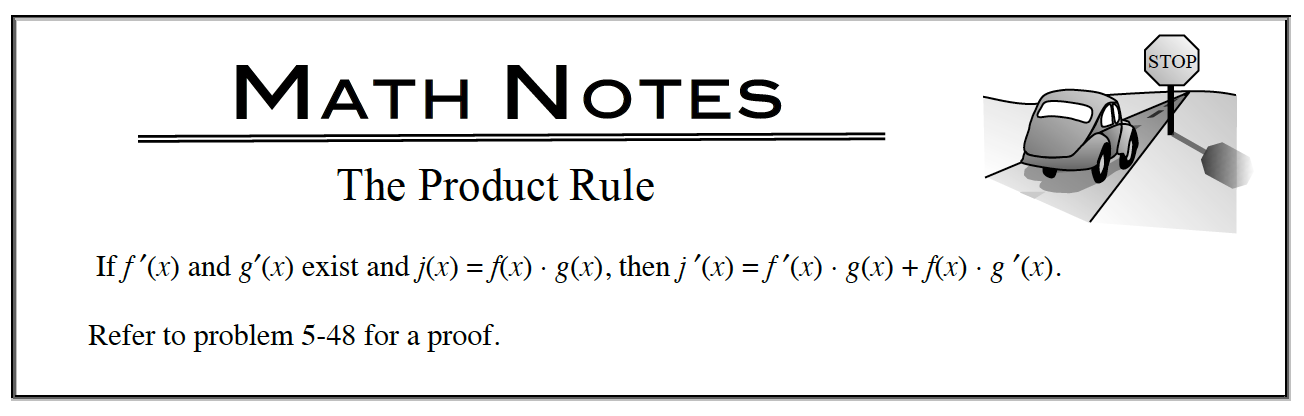### Home > APCALC > Chapter 5 > Lesson 5.2.1 > Problem5-52

5-52.

Differentiate each function if possible. If the function is one you cannot differentiate yet, say so and explain how you know. Be sure to use the appropriate notation. Homework Help ✎

You have the skills to find the derivative of five out of six of the problems below.

1. $y=x^{100}+100x$

Power Rule.

2. $H(t)=t^{-4}−6\sqrt{x}$

Before differentiating, rewrite the square root with a fractional exponent.

3. $y = x · 2^x$

Do you know how to find a derivative of 2x?

4. $y = x^8 \operatorname{cos}(x)$5. $f (x) = x^5(x^3 + π)$

Distribute first. Or use the Product Rule.

6. $y = \large\frac { 7 } { x } - \frac { x } { 7 }$

Before differentiating, rewrite the first fraction with exponents.